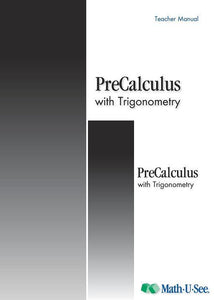or 6 weekly interest-free payments from \$14.83 withwhat's this?Demme Learning

# Math.U.See Pre Calculus with Trigonometry

Regular price \$20.00 \$51.11 Unit price per
Tax included. Shipping calculated at checkout.

## Math.U.See Pre Calculus with Trigonometry

#### Trigonometry, identities, polar equations, logarithms, sequences, limits and other topics to prepare for calculus.

Major Concepts and Skills Include:

• Understanding and working with trigonometric ratios, their reciprocals, and their inverses
• Working with trigonometric expressions and identities
• Understanding and working with polar coordinates and radian measure
• Graphing and analyzing trigonometric functions
• Understanding functions, limits, domain, and range
• Working with Arithmetic and Geometric Series and Sequences

• Understanding and applying the Laws of Sines and Cosines
• Understanding and applying the Sum, Difference, Double-Angle, and Half-Angle Identities
• Proving trigonometric identities
• Working with vectors and adding linear functions
• Understanding and working with logarithms
• Working with Euler's number and natural logarithms

PreCalculus Instruction Pack includes: Instruction Manual & DVD

PreCalculus 2 Student pack includes: Student Workbook & Test & Activity booklet.

Honors textbook: Provides extra practice & extension work

Grab an extra Student Pack for an additional Student.